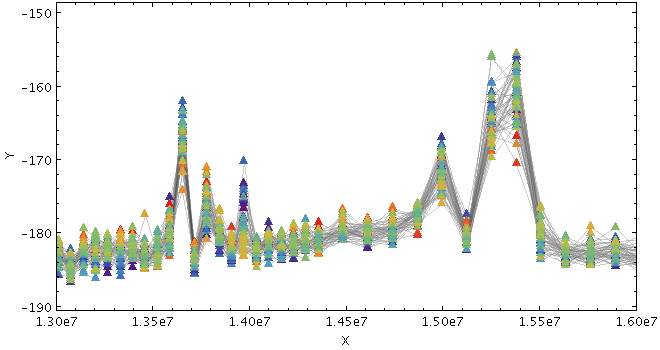Next Previous Up Contents
Next: yerrors
Up: Layer Types
Previous: lines

#### 8.3.16 `marks`

Plots N markers for each input row, with the X and Y coordinate values each supplied by an N-element array value.

Usage Overview:

```   layerN=marks shapeN=filled_circle|open_circle|... sizeN=<pixels>
xsN=<array-expr> ysN=<array-expr> inN=<table> ifmtN=<in-format>
istreamN=true|false icmdN=<cmds>
```

All the parameters listed here affect only the relevant layer, identified by the suffix `N`.

Example:```   stilts plot2plane in=LRS_NPW_V010_20071101.cdf xs=param\$frequency ys=RX2
xmin=13e6 xmax=16e6 xpix=660 icmd='head 50' auxmap=sron auxvisible=false
```

`icmdN = <cmds>`       (ProcessingStep[])
Specifies processing to be performed on the layer N input table as specified by parameter `inN`. The value of this parameter is one or more of the filter commands described in Section 6.1. If more than one is given, they must be separated by semicolon characters (";"). This parameter can be repeated multiple times on the same command line to build up a list of processing steps. The sequence of commands given in this way defines the processing pipeline which is performed on the table.

Commands may alteratively be supplied in an external file, by using the indirection character '@'. Thus a value of "`@filename`" causes the file `filename` to be read for a list of filter commands to execute. The commands in the file may be separated by newline characters and/or semicolons, and lines which are blank or which start with a '`#`' character are ignored.

`ifmtN = <in-format>`       (String)
Specifies the format of the input table as specified by parameter `inN`. The known formats are listed in Section 5.1.1. This flag can be used if you know what format your table is in. If it has the special value `(auto)` (the default), then an attempt will be made to detect the format of the table automatically. This cannot always be done correctly however, in which case the program will exit with an error explaining which formats were attempted. This parameter is ignored for scheme-specified tables.

[Default: `(auto)`]

`inN = <table>`       (StarTable)
The location of the input table. This may take one of the following forms:
• A filename.
• A URL.
• The special value "`-`", meaning standard input. In this case the input format must be given explicitly using the `ifmtN` parameter. Note that not all formats can be streamed in this way.
• A scheme specification of the form `:<scheme-name>:<scheme-args>`.
• A system command line with either a "`<`" character at the start, or a "`|`" character at the end ("`<syscmd`" or "`syscmd|`"). This executes the given pipeline and reads from its standard output. This will probably only work on unix-like systems.
In any case, compressed data in one of the supported compression formats (gzip, Unix compress or bzip2) will be decompressed transparently.
`istreamN = true|false`       (Boolean)
If set true, the input table specified by the `inN` parameter will be read as a stream. It is necessary to give the `ifmtN` parameter in this case. Depending on the required operations and processing mode, this may cause the read to fail (sometimes it is necessary to read the table more than once). It is not normally necessary to set this flag; in most cases the data will be streamed automatically if that is the best thing to do. However it can sometimes result in less resource usage when processing large files in certain formats (such as VOTable). This parameter is ignored for scheme-specified tables.

[Default: `false`]

`shadingN = auto|flat|translucent|transparent|density|aux|weighted <shade-paramsN>`       (ShapeMode)
Determines how plotted objects in layer N are coloured. This may be influenced by how many objects are plotted over each other as well as the values of other parameters. Available options (Section 8.4) are:
• `auto`
• `flat`
• `translucent`
• `transparent`
• `density`
• `aux`
• `weighted`
Each of these options comes with its own set of parameters to specify the details of how colouring is done.

[Default: `auto`]

`shapeN = filled_circle|open_circle|...`       (MarkerShape)
Sets the shape of markers that are plotted at each position of the scatter plot.

The available options are:

• `filled_circle`
• `open_circle`
• `cross`
• `x`
• `open_square`
• `open_diamond`
• `open_triangle_up`
• `open_triangle_down`
• `fat_circle`
• `fat_cross`
• `fat_x`
• `fat_square`
• `fat_diamond`
• `fat_triangle_up`
• `fat_triangle_up`
• `filled_square`
• `filled_diamond`
• `filled_triangle_up`
• `filled_triangle_down`

[Default: `filled_circle`]

`sizeN = <pixels>`       (Integer)
Size of the scatter plot markers. The unit is pixels, in most cases the marker is approximately twice the size of the supplied value.

[Default: `1`]

`xsN = <array-expr>`       (String)
Array giving the X coordinate array for each line.

The value is an array-valued algebraic expression based on column names as described in Section 10. Some of the functions in the Arrays class may be useful here.

`ysN = <array-expr>`       (String)
Array giving the Y coordinate array for each line.

The value is an array-valued algebraic expression based on column names as described in Section 10. Some of the functions in the Arrays class may be useful here.

Next Previous Up Contents
Next: yerrors
Up: Layer Types
Previous: lines

STILTS - Starlink Tables Infrastructure Library Tool Set